## A force of 100 N hangs in the center of a cable between two anchor points that are the same height off the ground and 30 m apart horizontall

Question

A force of 100 N hangs in the center of a cable between two anchor points that are the same height off the ground and 30 m apart horizontally. The object creates a sag of 2 m in the cable. Find the force exerted by each end of the cable on its anchor point.

in progress 0
6 months 2021-08-04T12:53:03+00:00 1 Answers 9 views 0

378.3N

Explanation:

According to Newton’s 3rd law, the force exerting on each anchor points would equal to the tension force T on each cable, which is created to support the 100N in the center

As the system remain balanced, then vertically the tension force would support the 100N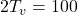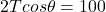where Θ is the angle between the cable and the vertical line intersecting the force F. As it’s 15m halfway between the 2 anchor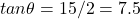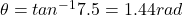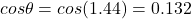therefore 2T0.132 = 100

T = 100/(2*0.132) = 378.3 N